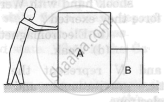Department of Pre-University Education, KarnatakaPUC Karnataka Science Class 11

# List All the Forces Acting on the Block B in Figure. - Physics

Short Note

List all the forces acting on the block B in figure.#### Solution

Forces acting on block B :

• A pair of electromagnetic forces between the blocks A and B.
• A pair of electromagnetic forces between the blocks B and the Earth.
• A pair of gravitational forces between the blocks B and the Earth.
• A pair of gravitational forces between the blocks A and B.
• A pair of action-reaction forces between the man and block A.
• Weight of the man, block A and block B as action forces on the ground in the downward direction.
• Reaction of the ground on the man, block A and block B in the upward direction to balance their weights.
Concept: Work Done by a Constant Force and a Variable Force
Is there an error in this question or solution?

#### APPEARS IN

HC Verma Class 11, Class 12 Concepts of Physics Vol. 1
Chapter 4 The Forces
Short Answers | Q 7 | Page 61
Share